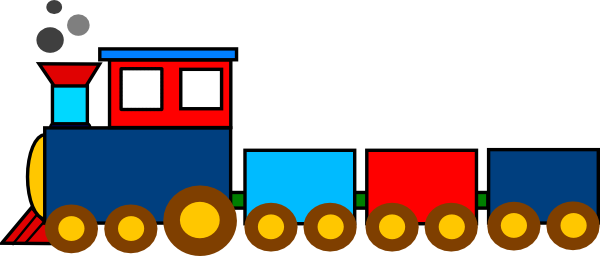### Examination Questions on Trains

#### Relative SpeedsRelative Speed of trains moving in the same direction = difference of their speedsRelative Speed of trains moving in the opposite direction = sum of their speeds

#### Convert kmph to metres per second and vice-versa.

Type the speed in kilometres per hour in the first box below OR
Type the speed in metres per second in the second box:

Kilometres per hour
equals
metres per second

To convert the speed in kmph into mps multiply the speed by 5/18 and to convert the speed in mps into kmph multiply by 18/5.

###### Click on the option you think is right and then check by clicking on the Show Answer button. You can change your option as many times as you like.

1. A train takes 9 seconds to cross a pole. If the speed of the train is 48 km/hr, the length of the train is

1. 150 m
2. 120 m
3. 90 m
4. 80 m

2. A train takes 10 seconds to cross a pole and 20 s to cross a platform of length 200 m. What is the length of the train?

1. 400 m
2. 300 m
3. 200 m
4. 100 m

3. A train 180 m long moving at the speed of 20 m/sec overtakes a man moving at a speed of 10 m/sec in the same direction. The train passed the man in:

1. 6 secs
2. 9 secs
3. 18 secs
4. 27 secs.

4. A train 280 m long is moving at a speed of 60 km/ph. What is the time taken by the train to cross a platform 220 m long?

1. 45 s
2. 40 s
3. 35 s
4. 30 s

5. Two trains each 200 m long move towards each other on parallel lines with velocities 20 kmph and 30 kmph respectively. What is the time that elapses when they first meet until they have cleared each other?

1. 20 s
2. 24.8 s
3. 28.8 s
4. 30 s

6. Two trains of lengths 100 m and 150 m are travelling in opposite directions at speeds of 75 kmph and 50 kmph respectively. What is the time taken by them to cross each other?

1. 7.4 s
2. 7.2 s
3. 7 s
4. 6.8 s

7. A train leaves station A at 5 A.M. and reaches station B at 9 A.M. on the same day. Another train leaves Station B at 7 A.M. and reaches station A at 10.30 A.M. on the same day. The time at which the two trains cross on another is

1. 7.56 A.M.
2. 8.26 A.M.
3. 8 A.M.
4. 7.36 A.M.

8. A train travelling with 5/7 of its actual speed covers 42 kms in 1 hr 40 mins 48 secs. Find the actual speed of the train.

1. 17 6/7 km/hr
2. 35 km/hr
3. 25 km/hr
4. 30 km/hr

9. A train crosses a telegraph post in 8 sec and a bridge 200 m long in 24 sec. What is the length of the train?

1. 100 m
2. 120 m
3. 140 m
4. 160 m